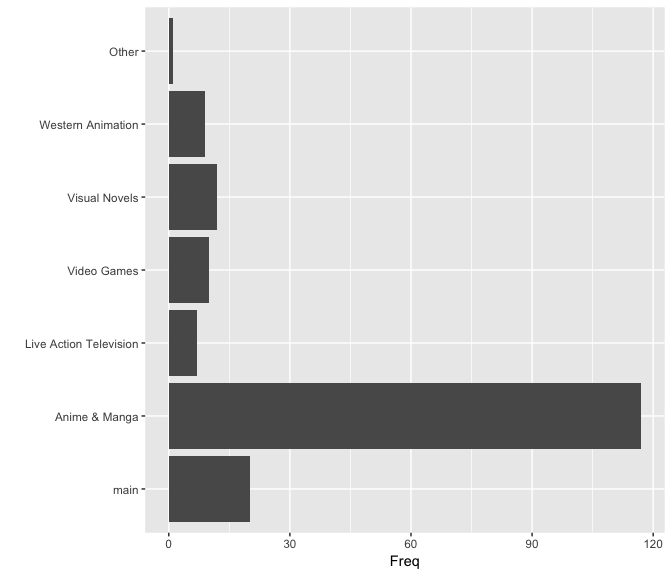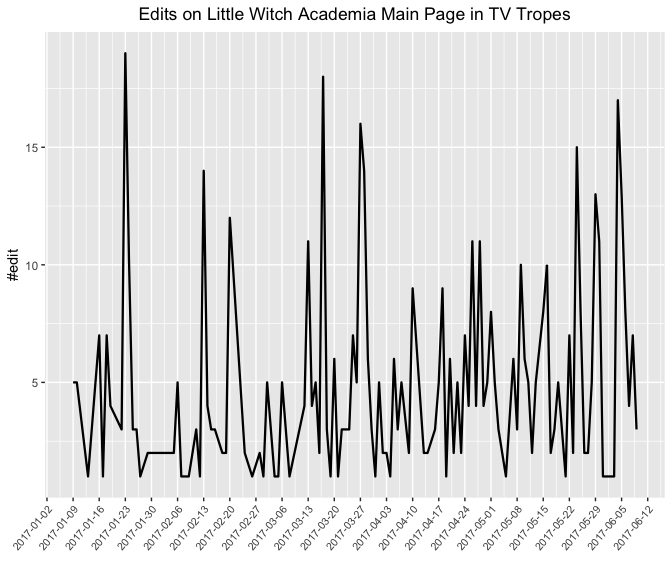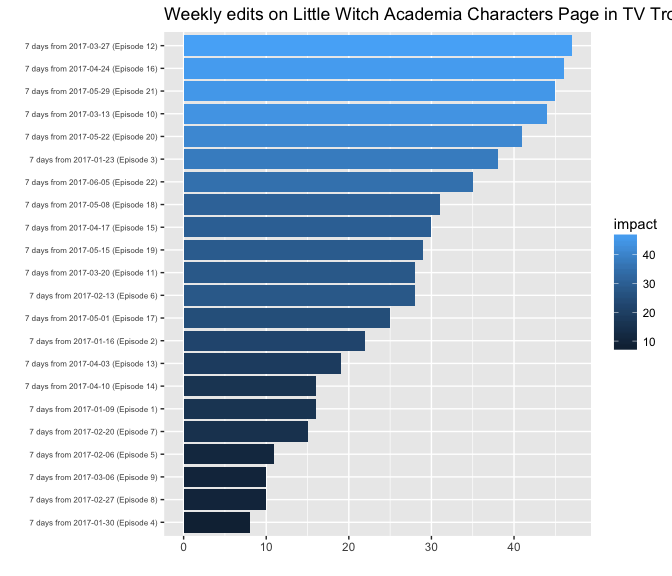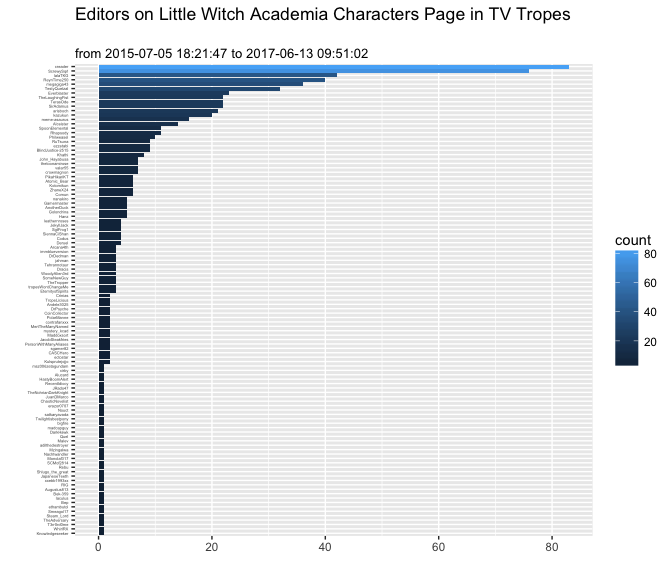# Quickstart

### Installation

Either you try stable CRAN version

install.packages("tropr")

Or unstable development version

devtools::install_github("zedoul/tropr")

You’ll need to use library to load as follows:

library(tropr)

### Get Content

library(tropr)

.url <- "http://tvtropes.org/pmwiki/pmwiki.php/Main/SenseiChan"
content <- trope_content(.url)
.df <- as.data.frame(content)
head(.df[, c("category", "link")])
##   category                                                           link
## 1     main          http://tvtropes.org/pmwiki/pmwiki.php/Main/HotTeacher
## 2     main                 http://tvtropes.org/pmwiki/pmwiki.php/Main/Moe
## 3     main         http://tvtropes.org/pmwiki/pmwiki.php/Main/CoolTeacher
## 4     main       http://tvtropes.org/pmwiki/pmwiki.php/Main/ChristmasCake
## 5     main http://tvtropes.org/pmwiki/pmwiki.php/Main/DudeWheresMyRespect
## 6     main        http://tvtropes.org/pmwiki/pmwiki.php/Main/OneOfTheKids

The output is tidy, so that you can easily turn them into a plot as follows:

library(ggplot2)
q <- ggplot(.df, aes(category)) + geom_bar() +
coord_flip() + ylab("Freq") + xlab("")
plot(q)### Cache trope data

It would be very hard to implement and to maintain data fetching module from the bottom. tropr provides easy-to-use function trope_cache which will check redirects, filtering, and data fetching automatically.

Here is one example of how you can use trope_cache.

library(tropr)

.urls <- c("http://tvtropes.org/pmwiki/pmwiki.php/Main/SenseiChan")
res <- trope_cache(.urls, depth = 3, filter_pattern = "/Main/")

It will fetch a set of tropes starting from .urls in three tree-depth. You can set filter option, where the example uses /Main/, to fetch specific category that you are interested in.

Once it cached, you can fetch those data with trope_data function, regardless of your network connection status. Note that cache directory is temporary given from tempdir() unless you set it by yourself with cache_dir parameter.

### Page history analysis

Apart from page analysis, tropr also provides functions for page history analysis. Here is the example with Little Witch Academia page history.

library(tropr)
library(ggplot2)
library(dplyr)

hist_content <- trope_history(.url)
.data <- aggr_history_daily_count(hist_content)
.resolution <- "1 week"

# In this case, we are interested in history only after the TV release, and
# before the episode 23
.data <- .data[c(47:169), ]

q <- ggplot(.data, aes(x = date, y = count)) +
geom_line(size= .8) +
theme(axis.text.x = element_text(angle = 50, hjust = 1, vjust = 1, size = 8),
plot.title = element_text(hjust = 0.5)) +
scale_x_date(date_breaks = .resolution) +
ggtitle("Edits on Little Witch Academia Main Page in TV Tropes") +
xlab("") + ylab("#edit")
plot(q)And we can compare the impact of episode 22, with others as follows:

library(tropr)
library(ggplot2)
library(magrittr)

impacts <- .data %>% group_by(date = cut(date, "week")) %>% summarise(impact = sum(count))
impacts$date <- paste0("7 days from ", as.character(impacts$date), " (", paste("Episode", rep(1:22)), ")")

q <- ggplot(impacts, aes(x = reorder(date, impact),
y = impact,
fill = impact)) +
geom_bar(stat= "identity") +
theme(axis.text.y = element_text(size = 6)) +
coord_flip() + ggtitle("Weekly edits on Little Witch Academia Characters Page in TV Tropes") +
ylab("") + xlab("")
plot(q)It is important to check the distribution of editors’ commitments on the page. Following example shows how you can do with tropr. Note that this will create distribution not based on subset like previous examples, but on whole dataset.

.data <- aggr_history_editor_count(hist_content)

q <- ggplot(.data, aes(x = reorder(editor, count),
y = count,
fill = count)) +
geom_bar(stat= "identity") +
theme(axis.text.y = element_text(size = 3)) +
coord_flip() + ggtitle("Editors on Little Witch Academia Characters Page in TV Tropes\n",
paste("from", tail(hist_content)[5, "datetime"],
"to", head(hist_content[1, "datetime"]))) +
ylab("") + xlab("")
plot(q)